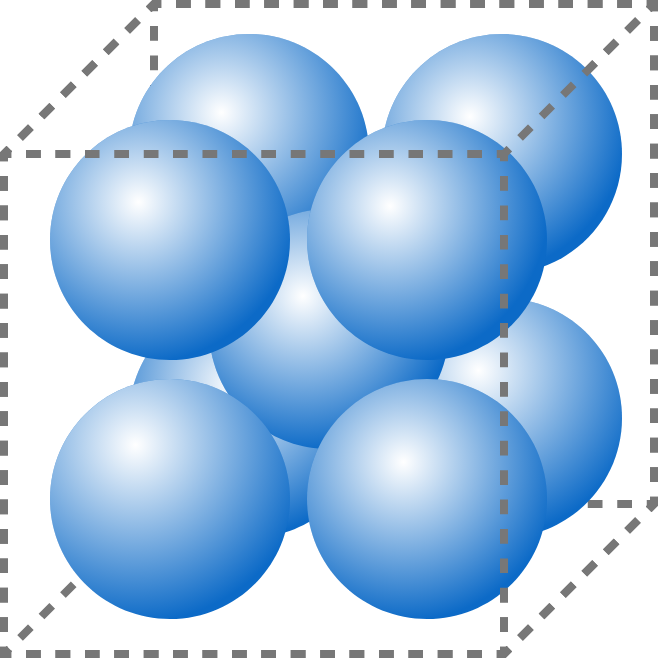Packing Nine Balls in a Cube

Geometry Level 39 identical, spherical balls are packed into a cube of edge length 100.

The 8 balls in corners are each tangent to the three faces making up its corner, and the $9^\text{th}$ ball is tangent to the other 8.

What is the radius of the spherical balls, to 1 decimal place?

×